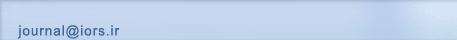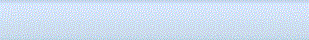[Home ] [Archive]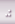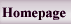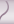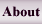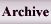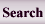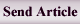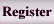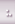Home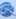Journal InformationArticles archiveSubmission InstructionRegistrationSubmit articleSite FacilitiesContact us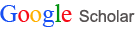All Since 2017 Citations 56124 18557 h-index 10 6 i10-index 13 4

Search in website
 Search the articlesSearch the site Advanced Search
 Enter your Email in the following box to receive the site news and information.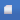Search published articles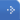Showing 132 results for Type of Study: Original

Ferenc Szidarovszky,
Volume 1, Issue 1 (5-2008)
Abstract

Abstract

Extended Oligopoly models will be introduced and examined in which the firms might face capacity limits, thresholds for minimal and maximal moves, and antitrust thresholds in the case of partial cooperation. Similar situation occurs when there is an additional cost of output adjustment, which is discontinuous at zero due to set-up costs. In these cases the payoff functions of the firms are nondifferentiable and in some cases even discontinuous. Under the usual concavity assumptions Cournot oligopolies have monotonic response functions and unique Cournot-Nash equilibrium. However the introduction of these more realistic additions into the oligopoly models creates a fundamentally new situation: the existence of no equilibrium or the presence of multiple, in some cases even infinitely many, equilibria. It also results in a very different asymptotic behavior of the dynamic extensions. The paper gives a brief survey of the relevant models, derives the response functions of the firms, and examines the existence and the number of equilibria. In the case of infinitely many equilibria the equilibrium-set will be also determined and characterized.

Emilio Spedicato, Marco Bonomi, Antonino Del Popolo,
Volume 1, Issue 1 (5-2008)
Abstract

Abstract

We consider an application of the ABS procedure to the linear systems arising from the primal-dual interior point methods where Newton method is used to compute path to the solution. When approaching the solution the linear system, which has the form of normal equations of the second kind, becomes more and more ill conditioned. We show how the use of the Huang algorithm in the ABS class can reduce the ill conditioning. Preliminary numerical experiments show that the proposed approach can provide a residual in the computed solution up to sixteen orders lower.

Volume 1, Issue 1 (5-2008)
Abstract

Abstract

We extend the concept of dynamic pricing by integrating it with “overselling with opportunistic cancellation” option, within the framework of dynamic policy. Under this strategy, to sell a stock of perishable product (or capacity) two prices are offered to customers at any given time period. Customers are categorized as high-paying and low-paying ones. The seller deliberately oversells its capacity if high paying customers show up, even when the capacity is already fully booked by low-paying customers. In that case, the sale to some low-paying customers is canceled, although an appropriate compensation must be provided. A dynamic programming approach is applied to formulate and solve this problem. We develop two models for continuous and periodic pricing, depending on the frequency of price changing. The advantage of this system over dynamic pricing model is investigated through some numerical examples. We also study some structural properties of the optimal policies.

Prof. Óbudai Galantai, Prof. Spedicato,
Volume 1, Issue 1 (5-2008)
Abstract

Abstract

This paper gives a survey of the theory and practice of nonlinear ABS methods including various types of generalizations and computer testing. We also show three applications to special problems, two of which are new.

Thomas L. Saaty,
Volume 1, Issue 1 (5-2008)
Abstract

Abstract

The Analytic Network Process (ANP) is a generalization of the Analytic Hierarchy Process (AHP). The basic structure is an influence network of clusters and nodes contained within the clusters. Priorities are established in the same way they are in the AHP using pairwise comparisons and judgment. Many decision problems cannot be structured hierarchically because they involve the interaction and dependence of higher-level elements in a hierarchy on lower-level elements. Not only does the importance of the criteria determine the importance of the alternatives as in a hierarchy, but also the importance of the alternatives themselves determines the importance of the criteria. Feedback enables us to factor the future into the present to determine what we have to do to attain a desired future. To illustrate ANP, one example is also presented.

Volume 2, Issue 1 (4-2010)
Abstract

The optimal harvesting policy is calculated as a function of the entering stock, the price state, the harvesting cost, and the rate of interest in the capital market. In order to determine the optimal harvest schedule, the growth function and stumpage price process are estimated for the Swedish mixed species forests. The stumpage price is assumed to follow a stochastic Markov process. A stochastic dynamic programming technique and traditional deterministic methods are used to obtain the optimal decisions. The expected present value of all future profits is maximized. The results of adaptive optimization are compared with results obtained by the traditional deterministic approach. The results show a significant increase in the expected economic values via optimal adaptive decisions.

Jain, Lachhwani,
Volume 2, Issue 1 (4-2010)
Abstract

We develop an algorithm for the solution of multiobjective linear plus fractional programming problem (MOL+FPP) when some of the constraints are homogeneous in nature. Using homogeneous constraints, first we construct a transformation matrix T which transforms the given problem into another MOL+FPP with fewer constraints. Then, a relationship between these two problems, ensuring that the solution of the original problem can be recovered from the solution of the transformed problem, is established. We repeat this process of transformation until all the homogeneous constraints are removed. Then, we discuss the multi objective programming part, for which fuzzy programming methodology is proposed which works for the minimization of perpendicular distances between two hyper planes (curves) at the optimal points of the objective functions. A suitable membership function is defined with the help of the supremum perpendicular distance. A compromised optimal solution is obtained as a result of the minimization of the The supremum perpendicular distance. The corresponding optimal solution to the original problem is obtained using the transformation matrix. Finally, an example is given to illustrate the proposed model.

Volume 2, Issue 1 (4-2010)
Abstract

We suggest a method for finding the non-dominated points of the production possibility set (PPS) with variable returns to scale (VRS) technology in data envelopment analysis (DEA). We present a multiobjective linear programming (MOLP) problem whose feasible region is the same as the PPS under variable returns to scale for generating non-dominated points. We demonstrate that Pareto solutions of the MOLP produce efficient units in DEA, and vice versa. We solve the MOLP problem by using a finite number of weights which are extreme rays of the cone generated by the efficient solutions. We obtain new efficient points by changing weights, and thus the efficient solutions set is produced.

Jain,
Volume 2, Issue 1 (4-2010)
Abstract

The fuzzy approach has undergone a profound structural transformation in the past few decades. Numerous studies have been undertaken to explain fuzzy approach for linear and nonlinear programs. While, the findings in earlier studies have been conflicting, recent studies of competitive situations indicate that fractional programming problem has a positive impact on comparative scenario. We propose one of the best interval approximations, close interval approximation of piecewise quadratic fuzzy numbers for solving fuzzy number fractional programming problem without converting it to a crisp problem. A new form of simplex method is introduced here for solving fuzzy number fractional programming problem using fuzzy arithmetic. The fuzzy analogue of some important theorems of fuzzy fractional programming problem proved. A fuzzy fractional programming problem is worked out as an example to illustrate the proposed method.

Volume 2, Issue 2 (6-2011)
Abstract

We consider the maximal covering location-allocation problem with multiple servers. The objective is to maximize the population covered, subject to constraints on the number of service centers, total number of servers in all centers, and the average waiting time at each center. Each center operates as an M/M/k queuing system with variable number of servers. The total costs of establishing centers and locating servers should not exceed a predetermined amount. We present a mathematical model for the problem, and propose a heuristic solution procedure with two local search algorithms for improving the solutions. Finally, some computational results are presented.

Volume 2, Issue 2 (6-2011)
Abstract

A novel optimal single machine replacement policy using a single as well as a two-stage decision making process is proposed based on the quality of items produced. In a stage of this policy, if the number of defective items in a sample of produced items is more than an upper threshold, the machine is replaced. However, the machine is not replaced if the number of defective items is less than a lower threshold. Nonetheless, when the number of defective item falls between the upper and the lower thresholds, the decision making process continues inspecting and possibly repairing the machine and the decision making process goes on to collect more samples. The primary objective of own work is to determine the optimal values of both the upper and the lower thresholds using a Markov process to minimize the total cost associated with a machine replacement policy.

Salahi,
Volume 2, Issue 2 (6-2011)
Abstract

Semidefinite optimization relaxations are among the widely used approaches to find global optimal or approximate solutions for many nonconvex problems. Here, we consider a specific quadratically constrained quadratic problem with an additional linear constraint. We prove that under certain conditions the semidefinite relaxation approach enables us to find a global optimal solution of the underlying problem in polynomial time .

Mahdavi, Paydar, Solimanpur,
Volume 2, Issue 2 (6-2011)
Abstract

A fuzzy goal programming-based approach is used to solve a proposed multi-objective linear programming model and simultaneously handle two important problems in cellular manufacturing systems, viz. cell formation and layout design. Considerations of intra-cell layout, the intra-cell material handling can be calculated exactly. The advantages of the proposed model are considering machining cost, inter-cell, intra-cell (forward and backward) material handling, operation sequence and resource constraints on the capacity of machines. To illustrate applicability of the proposed model, an example is solved and computational results are noted.

Valliathal, Uthayakumar,
Volume 2, Issue 2 (6-2011)
Abstract

We discuss the effects of inflation and time discounting on an EOQ model for deteriorating items under stock-dependent demand and time-dependent partial backlogging. The inventory model is studied under the replenishment policy starting with no shortages. We then use MATLAB to find the optimal replenishment policies. The objective of this model is to maximize the total profit (TP) which includes the sales revenue, purchase cost, the set up cost, holding cost, shortage cost and opportunity cost due to lost sales. Analytical results are given to justify the model. Finally, numerical examples are presented to determine the developed model and the solution procedure. Sensitivity analysis of the optimal solution with respect to major parameters is carried out. We propose a solution procedure to find the solution and obtain some managerial results by using sensitivity analysis.

Sheibani,
Volume 2, Issue 2 (6-2011)
Abstract

We describe a hybrid meta-heuristic algorithm for combinatorial optimization problems with a specific reference to the travelling salesman problem (TSP). The method is a combination of a genetic algorithm (GA) and greedy randomized adaptive search procedure (GRASP). A new adaptive fuzzy a greedy search operator is developed for this hybrid method. Computational experiments using a wide range of standard benchmark problems indicate that the proposed hybrid meta-heuristic approach is very efficient.

Fathali, Zaferanieh,
Volume 2, Issue 2 (6-2011)
Abstract

We consider the two well knownminimax and minisum single facility location problems in the plane which has been divided into two regions, and by a straight line. The two regions are measured by various norms . We focus on three special cases in which the regions and are measured by and norms, and block norms, two distinct block norms. Based on the properties of block norms then we use linear or almost linear problems in different cases to achieve the optimal solution.

Volume 3, Issue 1 (4-2012)
Abstract

We develop an optimization model based on Markovian approach to determine the optimum value of thresholds in a proposed acceptance sampling design. Consider an acceptance sampling plan where items are inspected and when the number of conforming items between successive defective items falls below a lower control threshold value, then the batch is rejected, and if it falls above a control threshold value, then the batch is accepted and if it falls within the thresholds, the process of inspecting the items continues. A decision is made to accept or reject the batch. We begin with developing a Markov model for determining performance measures of sampling designs, resulting in an acceptance sampling plan optimized based on the minimum angle method. Then, the performance measures of the acceptance sampling plan are determined and the optimum values of thresholds are selected in order to optimize the objective functions. In order to demonstrate the application of the proposed methodology, numerical examples are illustrated.
Etebari, Aaghaie, Khoshalhan,
Volume 3, Issue 1 (4-2012)
Abstract

In recent years, enriching traditional revenue management models by considering the customer choice behavior has been a main challenge for researchers. The terminology for the airline application is used as representative of the problem. A popular and an efficient model considering these behaviors is choice-based deterministic linear programming (CDLP). This model assumes that each customer belongs to a segment, which is characterized by a consideration set, which is a subset of the products provided by the firm that a customer views as options. Initial models consider a market segmentation, in which each customer belongs to one specific segment. In this case, the segments are defined by disjoint consideration sets of products. Recent models consider the extension of the CDLP to the general case of overlapping segments. The main difficulty, from a computational standpoint, in this approach is solving the CDLP efficiently by column generation. Indeed, it turns out that the column generation subproblem is difficult on its own. It has been shown that for the case of nonoverlapping segments, this can be done in polynomial time. For the more general case of overlapping segments, the column generation sub-problem is NP-hard for which greedy heuristics are proposed for computing approximate solutions. Here, we present a new approach to solve this problem by using a genetic algorithm and compare it with the column generation method. We comparatively investigate the effect of using the new approach for firm’s revenue